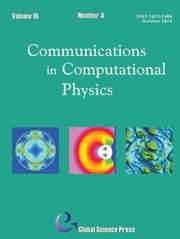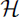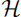Home
Hostname: page-component-55597f9d44-qcsxw Total loading time: 0.324 Render date: 2022-08-11T12:23:08.978Z Has data issue: true Feature Flags: { "shouldUseShareProductTool": true, "shouldUseHypothesis": true, "isUnsiloEnabled": true, "useRatesEcommerce": false, "useNewApi": true } hasContentIssue trueCommunications in Computational Physics

# On the Construction of Well-Conditioned Hierarchical Bases for(div)-Conforming ℝn Simplicial Elements

Published online by Cambridge University Press:  03 June 2015

*
Corresponding author.Email:wcai@uncc.edu

## Abstract

Hierarchical bases of arbitrary order for(div)-conforming triangular and tetrahedral elements are constructed with the goal of improving the conditioning of the mass and stiffness matrices. For the basis with the triangular element, it is found numerically that the conditioning is acceptable up to the approximation of order four, and is better than a corresponding basis in the dissertation by Sabine Zaglmayr [High Order Finite Element Methods for Electromagnetic Field Computation, Johannes Kepler Universität, Linz, 2006]. The sparsity of the mass matrices from the newly constructed basis and from the one by Zaglmayr is similar for approximations up to order four. The stiffness matrix with the new basis is much sparser than that with the basis by Zaglmayr for approximations up to order four. For the tetrahedral element, it is identified numerically that the conditioning is acceptable only up to the approximation of order three. Compared with the newly constructed basis for the triangular element, the sparsity of the mass matrices from the basis for the tetrahedral element is relatively sparser.

## Keywords

Type
Research Article
Information
Communications in Computational Physics , September 2013 , pp. 621 - 638

## Access options

Get access to the full version of this content by using one of the access options below. (Log in options will check for institutional or personal access. Content may require purchase if you do not have access.)

## References

Brezzi, F. and Fortin, M., Mixed and Hybrid Finite Element Methods, Springer Series in Computational Mathematics, 15. Springer-Verlag, New York, 1991.CrossRefGoogle Scholar
Roberts, J. E. and Thomas, J.-M., Mixed and Hybrid Methods, in Handbook of Numerical Analysis (Edited by Ciarlet, P. G. and Lions, J.-L.), Vol. II, pp. 523639, North-Holland, Amsterdam, 1991.Google Scholar
Love, A. E. H., A Treatise on the Mathematical Theory of Elasticity, 4th Ed., Dover Publication, New York, 1944.Google Scholar
Stratton, J. A., Electromagnetic Theory, McGraw-Hill, New York, 1941.Google Scholar
Temam, R., Navier-Stokes Equations: Theory and Numerical Analysis. With an appendix by Thomasset, F., 3rd Ed. Studies in Mathematics and Its Applications, 2. North-Holland Publishing, Amsterdam, 1984.Google Scholar
Cowling, T. G., Magnetohydrodynamics, Interscience Tracts on Physics and Astronomy, No. 4. Interscience Publishers, New York, 1957.Google Scholar
Raviart, P. A. and Thomas, J. M., A mixed finite element method for 2nd order elliptic problems, Mathematical aspects of finite element methods (Proc. Conf. Consiglio Naz. delle Ricerche (C.N.R.), Rome, 1975), pp. 292315. Lecture Notes in Math., Vol. 606, Springer, Berlin, 1977.CrossRefGoogle Scholar
Neédélec, J. C., Mixed finite elements in R3, Numer. Math., 35 (1980), pp. 315341.CrossRefGoogle Scholar
Neédélec, J. C., A new family of mixed finite elements in R3, Numer. Math., 50 (1986), pp. 57 81.CrossRefGoogle Scholar
Ciarlet, P. G., Mathematical Elasticity Vol. I. Three-dimensional Elasticity. Studies in Mathematics and Its Applications, 20. North-Holland Publishing, Amsterdam, 1988.Google Scholar
Brezzi, F., Douglas, J. Jr. and Marini, L. D., Two families of mixed finite elements for second order elliptic problems, Numer. Math., 47 (1985), pp. 217235.CrossRefGoogle Scholar
Brezzi, F., Douglas, J. Jr., Duraán, R. and Fortin, M., Mixed finite elements for second order elliptic problems in three variables, Numer. Math., 51 (1987), pp. 237250.CrossRefGoogle Scholar
Hiptmair, R., Canonical construction of finite elements, Math. Comp., 68 (1999), pp. 13251346.CrossRefGoogle Scholar
Hiptmair, R., Finite elements in computational electromagnetism, Acta Numer., 11 (2002), pp. 227339.CrossRefGoogle Scholar
Arnold, D. N., Falk, R. S. and Winther, R., Finite element exterior calculus, homological techniques, and applications, Acta Numer., 15 (2006), pp. 1155.CrossRefGoogle Scholar
Arnold, D. N., Falk, R. S. and Winther, R., Finite element exterior calculus: from Hodge theory to numerical stability, Bull. Amer. Math. Soc. (N.S.), 47 (2010), pp. 281354.CrossRefGoogle Scholar
Bluck, M. J., Conforming hierarchical basis functions, Commun. Comput. Phys., 12 (2012), pp. 12151256.CrossRefGoogle Scholar
Ainsworth, M. and Coyle, J., Hierarchic finite element bases on unstructured tetrahedral meshes, Internat. J. Numer. Methods Engrg., 58 (2003), pp. 21032130.CrossRefGoogle Scholar
Akin, J. E., Finite Elements for Analysis and Design, Academic Press, London, 1994.Google Scholar
Xin, J., Pinchedez, K. and Flaherty, J. E., Implementation of hierarchical bases in FEMLAB for simplicial elements, ACM Trans. Math. Software, 31 (2005), pp. 187200.CrossRefGoogle Scholar
Zaglmayr, S., High Order Finite Element Methods for Electromagnetic Field Computation, Ph.D. Dissertation, Johannes Kepler Universitaät, Linz, 2006.Google Scholar
Babusøka, I., Szabo, B. A. and Katz, I. N., The p-version of the finite element method, SIAM J. Numer. Anal., 18 (1981), pp. 515545.CrossRefGoogle Scholar
Babusøka, I. and Suri, M., The p and h-p versions of the finite element method, basic principles and properties, SIAM Rev., 36 (1994), pp. 578632.CrossRefGoogle Scholar
Carnevali, P., Morris, R. B., Tsuji, Y. and Taylor, G., New basis functions and computational procedures for p-version finite element analysis, Int. J.Num. Meth. Eng., 36 (1993), pp. 37593779.CrossRefGoogle Scholar
Adjerid, S., Aiffa, M. and Flaherty, J. E., Hierarchical finite element bases for triangular and tetrahedral elements, Comput. Methods Appl. Mech. Engrg., 190 (2001), pp. 29252941.CrossRefGoogle Scholar
Webb, J. P., Hierarchal vector basis functions of arbitrary order for triangular and tetrahedral finite elements, IEEE Trans. Antennas and Propagation, 47 (1999), pp. 12441253.CrossRefGoogle Scholar
Ainsworth, M. and Coyle, J., Hierarchic hp-edge element families for Maxwell’s equations on hybrid quadrilateral/triangular meshes, Comput. Methods Appl. Mech. Engrg., 190 (2001), pp. 67096733.CrossRefGoogle Scholar
Xin, J. and Cai, W., A well-conditioned hierarchical basis for triangular H(curl)-conforming elements, Commun. Comput. Phys., 9 (2011), pp. 780806.CrossRefGoogle Scholar
Xin, J., Guo, N. and Cai, W., On the construction of well-conditioned hierarchical bases for tetrahedral H(curl)-conforming Nédélec element, J. Comput. Math., 29 (2011), pp. 526542.CrossRefGoogle Scholar
Xin, J. and Cai, W., Well-conditioned orthonormal hierarchical L2 bases on Rn simplicial elements, J. Sci. Comput., 50 (2012), pp. 446461.CrossRefGoogle Scholar
Dunkl, C. F. and Xu, Y., Orthogonal Polynomials of Several Variables, Encyclopedia of Mathematics and its Applications, 81. Cambridge University Press, Cambridge, 2001.Google Scholar
Magnus, W., Oberhettinger, F. and Soni, R. P., Formulas and Theorems for the Special Functions of Mathematical Physics, Die Grundlehren der mathematischen Wissenschaften, Band 52, Springer-Verlag, New York, 3rd enlarged edition, 1966.Google Scholar
Dautray, R. and Lions, J.-L., Mathematical Analysis and Numerical Methods for Science and Technology, Vol. 3. Spectral Theory and Applications, Springer-Verlag, Berlin, 1990.Google Scholar
Kellogg, O. D., Foundations of Potential Theory, Reprint from the first edition of 1929. Die Grundlehren der Mathematischen Wissenschaften, Band 31, Springer-Verlag, New York, 1967.Google Scholar
Cockburn, B., Kanschat, G. and Schoötzau, D., A note on discontinuous Galerkin divergence-free solutions for the Navier-Stokes equations, J. Sci. Comput., 31 (2007), pp. 6173.CrossRefGoogle Scholar
Greif, C., Li, D., Schoötzau, D. and Wei, X., A mixed finite element method with exactly divergence-free velocities for incompressible magnetohydrodynamics, Comput. Methods Appl. Mech. Engrg., 199 (2010), pp. 28402855.CrossRefGoogle Scholar
4
Cited by

# Save article to Kindle

Note you can select to save to either the @free.kindle.com or @kindle.com variations. ‘@free.kindle.com’ emails are free but can only be saved to your device when it is connected to wi-fi. ‘@kindle.com’ emails can be delivered even when you are not connected to wi-fi, but note that service fees apply.

Find out more about the Kindle Personal Document Service.

On the Construction of Well-Conditioned Hierarchical Bases for(div)-Conforming ℝn Simplicial Elements Available formats × # Save article to Dropbox To save this article to your Dropbox account, please select one or more formats and confirm that you agree to abide by our usage policies. If this is the first time you used this feature, you will be asked to authorise Cambridge Core to connect with your Dropbox account. Find out more about saving content to Dropbox. On the Construction of Well-Conditioned Hierarchical Bases for(div)-Conforming ℝn Simplicial Elements
Available formats
×

# Save article to Google Drive

To save this article to your Google Drive account, please select one or more formats and confirm that you agree to abide by our usage policies. If this is the first time you used this feature, you will be asked to authorise Cambridge Core to connect with your Google Drive account. Find out more about saving content to Google Drive.

On the Construction of Well-Conditioned Hierarchical Bases for(div)-Conforming ℝn Simplicial Elements
Available formats
×
×